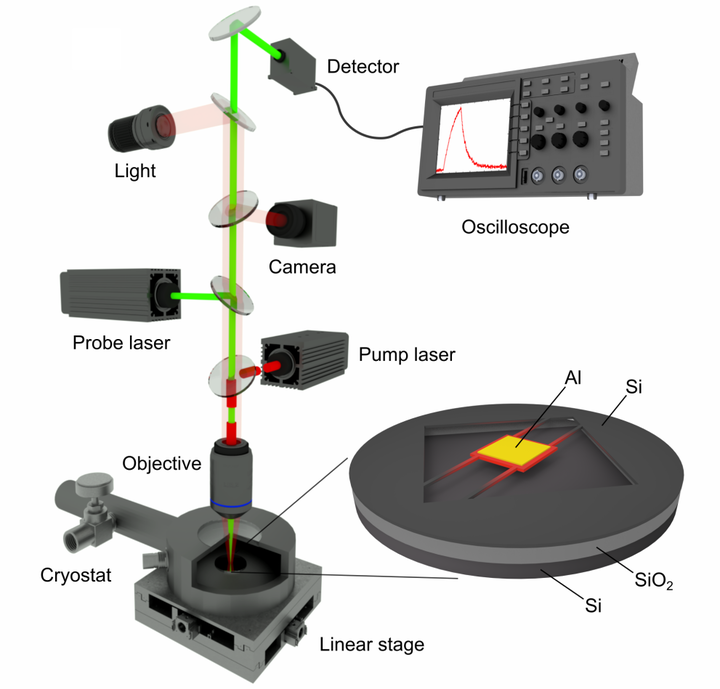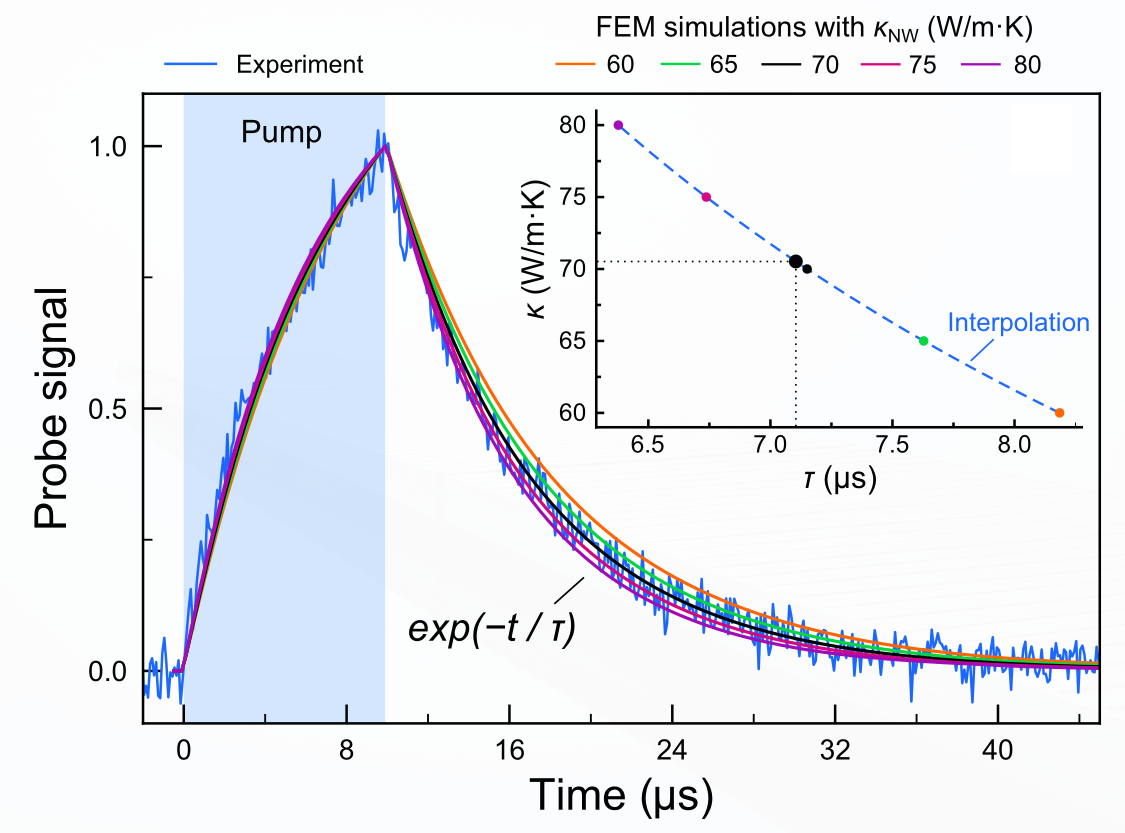# Micro time-domain thermoreflectanceMicro time-domain thermoreflectance (μTDTR) is a contactless optical pump-probe method for local thermal measurements of nanostructures. It enables measurements of thermal decay time through a structure of interest and extracting the thermal conductivity of the structure.

## Working principle

Sthe schematic of the μTDTR setup is shown above. The sample is placed in a cryostat at a high vacuum to avoin convection. Pulses of the pump laser are periodically heating a metal pad in the center of the sample, while the continuous-wave probe laser monitors the temperature of the metal pad using the thermoreflectance effect. Each pulse of the pump laser causes a rise in the temperature of the metal pad. The temperature rise and subsequent cooling are monitored by the probe laser reflected from the pad and directed into a detector connected to an oscilloscope. The oscilloscope integrates the signals over the thousands of pulses to obtain a smooth cooling curve shown below.

## Thermal conductivity extraction

The cooling curve follows $\exp(-t/\tau)$, where $\tau$ is the characteristic thermal decay time of the measured sample. To extract the thermal conductivity, the experimentally measured $\tau$ is compared to the curves obtained using finite element method (FEM) simulations of the same sample, as shown in the figure below.In the simulations, the thermal conductivity of the measured nanostructures acts as a free parameter that enables roughly reproducing the experimentally measured curve. Then, our algorithm interpolates the obtained dependence of the thermal conductivity on the decay time and finds the value that corresponds precisely to the measured decay time, as shown in inset of the figure above.

## Acknowledgments

This experimental technique has been developed in Nomura lab at the University of Tokyo. More details about this method can be found in the following publications: